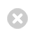Close

MPHY0021: Research Software Engineering With Python

Home### Setup for this notebook¶

We need to use a metaprogramming trick to make this teaching notebook work. I want to be able to put explanatory text in between parts of a class definition, so I'll define a decorator to help me build up a class definition gradually.

In :
def extend(class_to_extend):
""" Metaprogramming to allow gradual implementation
of class during notebook. Thanks to
http://www.ianbicking.org/blog/2007/08/opening-python-classes.html """
def decorator(extending_class):
for name, value in extending_class.__dict__.items():
if name in ['__dict__', '__module__', '__weakref__', '__doc__']:
continue
setattr(class_to_extend, name, value)
return class_to_extend
return decorator


Imagine we wanted to make a library to describe some kind of symbolic algebra system:

In :
class Term:
def __init__(self, symbols=[], powers=[], coefficient=1):
self.coefficient = coefficient
self.data={symbol: exponent for symbol,exponent
in zip(symbols, powers)}

In :
class Expression:
def __init__(self, terms):
self.terms = terms


So that $5x^2y+7x+2$ might be constructed as:

In :
first = Term(['x', 'y'], [2, 1], 5)

second = Term(['x'], , 7)

third = Term([], [], 2)

result = Expression([first, second, third])


This is pretty cumbersome.

What we'd really like is to have 2x+y give an appropriate expression.

First, we'll define things so that we can construct our terms and expressions in different ways.

In :
class Term:
def __init__(self, *args):
# Copy constructor
self.from_symbol(*args)
self.from_dictionary(*args)
else:
self.from_lists(*args)

def from_constant(self, constant):
self.coefficient = constant
self.data = {}

def from_symbol(self, symbol, coefficient=1, power=1):
self.coefficient = coefficient
self.data = {symbol: power}

def from_dictionary(self, data, coefficient=1):
self.data = data
self.coefficient = coefficient

def from_lists(self, symbols=[], powers=[], coefficient=1):
self.coefficient = coefficient
self.data={symbol: exponent for symbol,exponent
in zip(symbols, powers)}

In :
class Expression:
def __init__(self, terms=[]):
self.terms = list(terms)


We could define add() and multiply() operations on expressions and terms:

In :
@extend(Term)
class Term:
return Expression((self,) + others)


In :
@extend(Term)
class Term:
def multiply(self, *others):
result_data = dict(self.data)
result_coeff = self.coefficient
# Convert arguments to Terms first if they are
# constants or integers
others = map(Term, others)

for another in others:
for symbol, exponent in another.data.items():
if symbol in result_data:
result_data[symbol] += another.data[symbol]
else:
result_data[symbol] = another.data[symbol]
result_coeff *= another.coefficient

return Term(result_data, result_coeff)

In :
@extend(Expression)
class Expression:
result = Expression(self.terms)

for another in others:
if type(another) == Term:
result.terms.append(another)
else:
result.terms += another.terms

return result


We can now construct the above expression as:

In :
x = Term('x')
y = Term('y')

first = Term(5).multiply(Term('x'), Term('x'), Term('y'))
second = Term(7).multiply(Term('x'))
third = Term(2)


This is better, but we still can't write the expression in a 'natural' way.

However, we can define what * and + do when applied to Terms!:

In :
@extend(Term)
class Term:

def __mul__(self, other):
return self.multiply(other)

In :
@extend(Expression)
class Expression:
def multiply(self, another):
# Distributive law left as exercise
pass


In :
x_plus_y = Term('x') + 'y'
x_plus_y.terms

Out:
'y'
In :
five_x_ysq = Term('x') * 5 * 'y' * 'y'

print(five_x_ysq.data, five_x_ysq.coefficient)

{'x': 1, 'y': 2} 5


This is called operator overloading. We can define what add and multiply mean when applied to our class.

Note that this only works so far if we multiply on the right-hand-side! However, we can define a multiplication that works backwards, which is used as a fallback if the left multiply raises an error:

In :
@extend(Expression)
class Expression:

In :
@extend(Term)
class Term:
def __rmul__(self, other):
return self.__mul__(other)


In :
5 * Term('x')

Out:
<__main__.Term at 0x7f26b8a3a460>

It's not easy at the moment to see if these things are working!

In :
fivex = 5 * Term('x')
fivex.data, fivex.coefficient

Out:
({'x': 1}, 5)

We can add another operator method __str__, which defines what happens if we try to print our class:

In :
@extend(Term)
class Term:
def __str__(self):
def symbol_string(symbol, power):
if power == 1:
return symbol
else:
return f"{symbol}^{power}"

symbol_strings=[symbol_string(symbol, power)
for symbol, power in self.data.items()]

prod = '*'.join(symbol_strings)

if not prod:
return str(self.coefficient)
if self.coefficient == 1:
return prod
else:
return f"{self.coefficient}*{prod}"

In :
@extend(Expression)
class Expression:
def __str__(self):
return '+'.join(map(str, self.terms))

In :
first = Term(5) * 'x' * 'x' * 'y'
second = Term(7) * 'x'
third = Term(2)
expr = first + second + third

In :
print(expr)

5*x^2*y+7*x+2